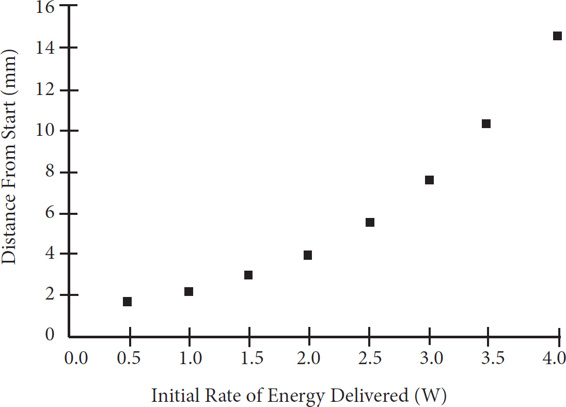# SAT Math Multiple Choice Question 150: Answer and Explanation

### Test Information

Question: 150

15. Mr. Lastorka's science class is running experiments with an energy-efficient model electric car. As the initial rate of energy delivered to the car, measured in watts, increases, the number of millimeters moved by the car from its starting position increases exponentially. The results of several trial runs are shown on the scatterplot graph below.Based on the data, the students in Mr. Lastorka's class determine the exact equation involving Watts, x, and total distance from start, y . They call the function y = f(x). Mr. Lastorka then instructs his class to reflect y = f(x) over the x-axis. He challenges each student to determine the new function and what it would mean from a physics perspective. Four student pairs gave their answers below. Who is correct, and for what reasons?

• A. Charles and Shannon, who identify the new equation as y = -2x and explain that the new graph indicates that the car is still moving forward at the same rate as before.
• B. Michael and Lauren, who identify the new equation as y = -2x and explain that the new graph indicates the car is now moving in reverse at the same rate as before.
• C. Matthew and Karen, who identify the new equation as y = 2-x and explain that the new graph indicates that the car is now moving forward more rapidly than before.
• D. Andy and Joanie, who identify the new equation as y = 2-x and explain that the new graph indicates that the car is no longer moving in any direction.